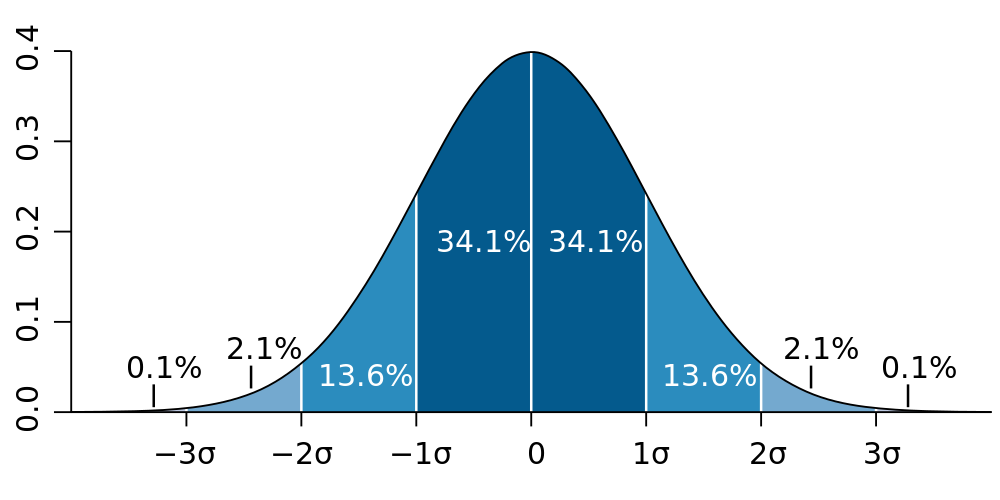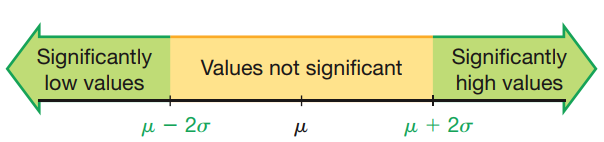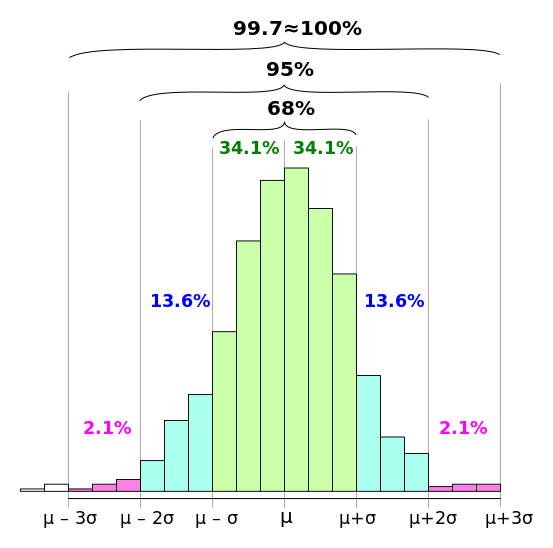# Measures of center and measures of spread part II

02/11/2020

## Instructions:

Use the left and right arrow keys to navigate the presentation forward and backward respectively. You can also use the arrows at the bottom right of the screen to navigate with a mouse.

FAIR USE ACT DISCLAIMER:
This site is for educational purposes only. This website may contain copyrighted material, the use of which has not been specifically authorized by the copyright holders. The material is made available on this website as a way to advance teaching, and copyright-protected materials are used to the extent necessary to make this class function in a distance learning environment. The Fair Use Copyright Disclaimer is under section 107 of the Copyright Act of 1976, allowance is made for “fair use” for purposes such as criticism, comment, news reporting, teaching, scholarship, education and research.

## Outline

• The following topics will be covered in this lecture:
• Range
• Standard deviation
• Chebyshev's Theorem
• Coefficient of variation

## Characteristics of dataCourtesy of M. W. Toews CC via Wikimedia Commons.

• Recall, we try to characterize data by a number of the features that it exhibits.
• Some of the key measures are:
1. Center: A representative value that indicates where the middle of the data set is located.
2. Variation: A measure of the amount that the data values vary.
3. Distribution: The nature or shape of the spread of the data over the range of values (such as bell-shaped).
4. Outliers: Sample values that lie very far away from the vast majority of the other sample values.
5. Time: Any change in the characteristics of the data over time.
• We will now begin studying measures of variation.
• There are several main measures of variation of a data set:
1. range;
2. standard deviation;
3. variance; and
4. coefficient of variation.
• We will discuss the meaning of each of these measures in the following.

## Range

• The simplest measure of variation is the range, which measures the width of the data values.

• In general, this is the least important measurement of variation but it is easy to compute.
• Suppose we have samples $$x_1,\cdots, x_n$$ and

\begin{align} x_\text{max} = \max_i(x_i) & & x_\text{min} = \min_i(x_i) \end{align}

• Then the range is computed as

$\text{Range} = x_\text{max} - x_\text{min}$

• For example, if our samples are $$22, 22, 26,$$ and $$24$$ then

$\text{Range} = 26 - 22 = 4.0$

• Discuss with a neighbor: is the range resistant to outliers? Why or why not?

• A: the range is computed from the largest and smallest values alone, so that outliers can dramatically impact the value.
• For example, suppose we have the sample values $$0, 0, 0, 0, 0, 0, 0, 0, 0, 1000$$. The range will be 1000 due the outlier;

• in particular, this measure of variation doesn't give a clear picture of the spread of the data which is actually very concentrated.

## Standard deviation

• Standard deviation is probably the most important measure of the spread of data.
• There are many ways that we can analyze data with standard deviation, among which is Chebyshev’s theorem which we will see later.
• Suppose that we have samples $$x_1,x_2,\cdots, x_n$$, and let us denote the sample mean as $\overline{x} = \frac{\sum_{i=1}^n x_i}{n}.$
• Then, the sample standard deviation denoted $$s$$ is defined as, $s = \sqrt{\frac{\sum_{i=1}^n\left(x_i - \overline{x}\right)^2}{n-1}}$
• This formula can be understood as follows:
• In each term of the sum $$\sum_{i=1}^n\left({\color{blue} {x_i - \overline{x}}} \right)^{\color{red}2}$$, we see how much sample $$i$$ deviates from the mean.
• The square in this equation keeps the quantity positive.
• The numerator thus gives the total sum of square differences of each sample from the mean.
• The denominator divides by the number of total samples minus one;
• there are good mathematical reasons for this, but for now we will say that we take an average over $$n-1$$ because the sample mean required one calculation of a statistic of the samples already.
• Finally, we take a square root to make the standard deviation in the same units as the variable $$x$$ – without this, the standard deviation would still be in the units squared.

### Standard deviation continued

• Note, we could have considered a different way to measure the variation than standard deviation.

• Consider, if we want to measure the total deviation we could instead write this as,

$\sum_{i=1}^n \vert x_i - \overline{x}\vert$

• We could then divide this by the total number of observations, which gives

$\text{Mean absolute deviation} = \frac{\sum_{i=1}^n \vert x_i - \overline{x}\vert}{n}$

• This is a possible choice for a similar measure of the variation, but the main issue lies in that the absolute value is not an “algebraic operation”.

• If we want to make calculations or inferences based on the formula above, this will become very difficult and there are few tools that work well with this statistic.

• For this reason, using the square as in the sample standard deviation

$s = \sqrt{\frac{\sum_{i=1}^n\left(x_i - \overline{x}\right)^2}{n-1}}$

we get a similar result, but one that is mathematically easier to manipulate.

### Standard deviation continued

• Note – the sample standard deviation can be computed equivalently as follows.
• Supposing again that we have samples $$x_1, \cdots, x_n$$, we can compute $s = \sqrt{\frac{n \left(\sum_{i=1}^n x_i^2 \right) - \left(\sum_{i=1}^n x_i\right)^2}{n\left(n-1\right)}}$
• This formula is totally equivalent to the last one and just requires some algebraic manipulation to show that this is the case.
• Often, the above calculation is preferable because we do not need to pre-compute the sample mean.
• This is also the form that is usually preferred for computer software calculation of the sample standard deviation.
• We should note, the sample standard deviation is a statistic because it is computed from samples.
• We can also consider the population standard deviation.
• Suppose that there are $$N$$ total members in the population with corresponding measurement values $$x_1 ,\cdots, x_N$$.
• If we had access to the entire population, could compute the population mean as $\text{Population mean} = \mu = \frac{\sum_{i=1}^N x_i }{N}.$
• With respect to the population mean $$\mu$$ the population standard deviation is given as, $\text{Population standard deviation} = \sigma = \sqrt{\frac{\sum_{i=1}^N \left(x_i - \mu\right)^2}{N}}.$

### Standard deviation continued

• Usually, we will not have access to the entire population $$x_1, \cdots, x_N$$.
• Instead, we will only have some smaller number of samples $$x_1, \cdots, x_n$$ for $$n< N$$.
• Therefore, the formulas which we use most often are, $s = \sqrt{\frac{\sum_{i=1}^n\left(x_i - \overline{x}\right)^2}{n-1}}$
• or $s = \sqrt{\frac{n \left(\sum_{i=1}^n x_i^2 \right) - \left(\sum_{i=1}^n x_i\right)^2}{n\left(n-1\right)}}$
• but not $\sigma = \sqrt{\frac{\sum_{i=1}^N \left(x_i - \mu\right)^2}{N}}.$
• One key difference to remember is that for the sample standard deviation, we have a very different denominator than with the population standard deviation.

### Standard deviation example

• We will not focus on calculating the sample standard deviation manually in this course;

• however, to demonstrate the concept, we will consider it once here.
• Suppose we have the samples $$22, 22, 26$$ and $$24$$.

• We wish to compute how much deviation there is in the data from the sample mean, so we will begin by computing this value

$\overline{x} = \frac{22 + 22 + 26 + 24 }{4} = \frac{94}{4}=23.5$

• We now compute the raw deviation of each sample from the sample mean:

\begin{align} x_1 - \overline{x} =& 22 - 23.5 = -1.5\\ x_2 - \overline{x} =& 22 - 23.5 = -1.5\\ x_3 - \overline{x} =& 26 - 23.5 = 2.5\\ x_4 - \overline{x} =& 24 - 23.5 = 0.5\\ \end{align}

• Squaring each value, we obtain $$2.25, 2.25, 6.25, 0.25$$, so that

$s = \sqrt{\frac{\sum_{i=1}^4 \left(x_i - \overline{x}\right)^2}{3}} = \sqrt{\frac{11}{3}}\approx 1.9$

• This shows how the sample standard deviation can be computed, but we will want a few ways to interpret the value.

## Interpreting the standard deviationCourtesy of Mario Triola, Essentials of Statistics, 6th edition

• The first way that we can interpret the standard deviation is with a simple “range rule of thumb”.
• For many data sets, the majority of sample values (on the order of $$95\%$$) will lie within two standard deviations of the mean.
• For this reason, we find measured values to suprising / significant when they lie outside of two standard deviations.
• To find significant values we can use the range rule of thumb as follows:
• Significantly low – a value $$x$$ is significantly low when $x \leq \mu - 2 \sigma$
• Significantly high – a value $$x$$ is significantly high when $x \geq \mu + 2 \sigma$
• Not significant – a value $$x$$ is not significant when $\mu - 2 \sigma < x < \mu + 2 \sigma$
• Notice that we are using the population mean and standard deviation in the rule above;
• however, when we have a large number of representative samples the sample mean and standard deviation can be treated as “close enough” to the population parameters.
• In the case that we have sufficiently many representative samples, we can use the same rule of thumb in terms of $$\overline{x}, s$$ instead of $$\mu, \sigma$$ above.

### Interpreting the standard deviation continuedCourtesy of Mario Triola, Essentials of Statistics, 6th edition

• Suppose we are examining the height $$x$$ in inches of all students at UNR.
• We will suppose that we have an exact record on each student, so that the population mean and standard deviation are given as, \begin{align} \mu =67 & & \sigma=6 \end{align}.
• Suppose we find a student who has a height of $$x = 78$$ inches.
• Discuss with a neighbor: is this student significantly tall by the range rule? Why?
• We recall that $$2 \times \sigma = 12$$ and $$\mu = 67$$.
• Comparing, $$78 <\mu +2 \sigma = 79$$ so that this is not a significantly high value for a student’s height.
• Likewise, $$78 > \mu - 2 \sigma = 55$$, so that this is not a significantly low value.
• By the range rule of thumb, in this example someone needs to be at least 6 foot 7 inches or at most 4 foot 7 inches tall to be statistically significant.
• Another interpretation is that the vast majority of students will have height between 4 feet and 7 inches, and 6 feet and 7 inches.
• A height outside of this range does not occur very often and thus it is surprising or significant to observe.

### Estimating the standard deviation with the range rule

• As a very rough estimate, we can approximate the sample standard deviation with the range rule.

• This uses the range, which is very sensitive to outliers, so that we should be careful in using this approximation.
• The only time we should consider using this approximation is when we have no computer or calculator on hand, and need a quick “back-of-an-envelope” calculation.

• The range rule of thumb for estimating the standard deviation is given as

$s \approx \frac{\text{Range}}{4}$

• Suppose we have the samples $$22, 22, 26$$ and $$24$$ once again.

• The sample standard deviation of the data is $$\approx 1.9$$.

• Discuss with a neighbor: what is the range rule of thumb estimate for the sample standard deviation? Is this very accurate in this case?

• The range rule of thumb gives $$\frac{26 - 22}{4} = \frac{4}{4}=1$$, which is not that accurate.

• This shows that we should only consider this as a very loose approximation, and in practice we should compute the sample standard deviation directly whenever possible.

## Variance

• When we describe the amount of variation in data, it is commonly described as the dispersion or spread in the data.

• This idea is illustrated by, e.g., the range rule of thumb which tells us how concentrated the data is.
• The word variance also has a specific meaning in statistics and is another tool for describing the variation / dispersion / spread of the data.

• Suppose that the data has a population standard deviation of $$\sigma$$ and a sample standard deviation of $$s$$.

• Then, the data has a population variance of $$\sigma^2$$.

• Likewise, the data has a sample variance of $$s^2$$.

• Therefore, for either a population parameter or a sample statistic, the variance is the square of the standard deviation.

• Because of this, the variance has units which are the square of the original units.
• For example, measuring the heights of students in inches, the standard deviation is in the units inches.

• However, the variance is in the unit $$\text{inches}^2$$.

## Important properties of standard deviation and variance

• We should introduce a few key properties of the standard deviation and the variance:
1. Standard deviation and variance are always non-negative by construction. \begin{align} s &= \sqrt{\frac{\sum_{i=1}^n \left(x_i - \overline{x}\right)^2}{n-1}} \\ \sigma & = \sqrt{\frac{\sum_{i=1}^N \left(x_i - \mu\right )^2}{N}} \end{align}
2. Standard deviation and variance are only zero when all values are equal and larger values means there is more spread in the data.
3. However, the size of the standard deviation and variance is also sensitive to outliers, and they can become large with a few outliers present.
4. The sample variance is an unbiased estimator of the population variance.
• Sampling error will mean that we usually do not have a sample variance that is equal to the population variance.
• However, over all possible samples this should usually be close to the true value.
5. The sample standard deviation is a biased estimator of the population standard deviation.
• Over repeated resampling, the different estimates for the population standard deviation via the sample standard deviation tend to be too small.
• However, for large numbers of samples this bias of the sample standard deviation often being too small won’t have much practical effect.

## The empirical (68-95-99.7) ruleCourtesy of Melikamp CC via Wikimedia Commons

• Recall now the bell curve picture that we often consider – we will suppose we have a population that is distributed as a bell shape.
• We suppose that the population mean is $$\mu$$ and population standard deviation $$\sigma$$.
• We suppose that the histogram represents the sample data which is mostly bell-shaped, but is smaller than the population so it is not exact.
• The empirical rule is as follows:
• Approximately $$68\%$$ of the sample data will lie within one standard deviation $$\sigma$$ of the population mean $$\mu$$, i.e., in $[\mu - \sigma, \mu + \sigma].$
• Approximately $$95\%$$ of sample data will lie within two standard deviations $$2\sigma$$ of the population mean $$\mu$$, i.e., in $[\mu - 2\sigma, \mu + 2\sigma].$
• Approximately $$99.7\%$$ of sample data will lie within three standard deviations $$3\sigma$$ of the population mean $$\mu$$, i.e., in $[\mu - 3\sigma, \mu + 3\sigma].$
• This tells us that for normal data, the spread can be easily interpreted from the standard deviation.

### The empirical (68-95-99.7) rule exampleCourtesy of Melikamp CC via Wikimedia Commons

• Let us consider an example.
• IQ scores have a bell-shaped distribution with a mean of $$100$$ and a standard deviation of $$15$$.
• Discuss with a neighbor: what percentage of IQ scores are between $$70$$ and $$130$$?
• We should note that the range $$[70, 130]$$ is equivalent to $[\mu - 2 \sigma, \mu + 2\sigma].$
• Therefore, the empirical rule tells us that about $$95\%$$ of IQ scores to lie within this range.

## Chebyshev's Theorem

• A very similar rule is known as Chebyshev’s theorem:
The proportion (or fraction) of any set of data lying within $$K$$ standard deviations of of the mean is always at least $$1-\frac{1}{K^2}$$ where $$K>1$$.
• Discuss with a neighbor: suppose $$K=2$$, what does this statement tell us?
• For $$K=2$$, we say at least $1 - \frac{1}{2^2} = 1 - \frac{1}{4} = \frac{3}{4}$ of data lies within $$K=2$$ standard deviations.
• Note, this holds for any distribution whereas the empirical rule only holds for normal data.
• If we know the data is in fact normal, then $$95\% > 75\% =1 - \frac{1}{2^2}$$ lies within $$K=2$$ standard deviations.
• We note thus there are two major differences between Chebyshev’s theorem and the empricical rule:
1. The empirical rule only holds for normal data, while Chebyshev’s theorem holds for any type of data.
2. However, Chebyshev’s theorem is only a lower bound on at least how much data lies within standard deviations and is a much weaker statement on how much.